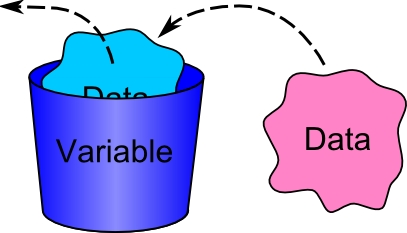PHP

# variables and output statements in PHPWe have 2  functions for output in PHP those are echo() and Print ()

actually output statements used to send output to the client (browser)

differences between echo() and print() are

1. echo() is faster than print() in execution
2. print() is return an integer value where echo() dosent return a value

in PHP the semicolon (;) is used to separate the two statements or scripts in PHP

In PHP, we use // or # to make a one-line comment

/* and */ to make a comment block:

## Variables in PHP

1. variables are used used to store values like text, string, numbers,arrays,objects, etc..
2. when a variable is set it can be used again and again in PHP program.
3. all the variables in PHP starts with \$.
4. in PHP an variable dose not need to be declare before it being set.
5. it is not necessary to tell PHP which data type is the variable.
6. PHP automatically converts the variable into correct data type depending on the situation where it was being used.

Example:

```<?php
\$a=”abc”;
\$b=”xyz”;
\$c=”\$a+\$b;
echo \$c;
?>```

output will be “abcxyz”

## Variable naming rules

1. A variable name can have alphanumeric characters and underscore “_”.  That is all alphabets and numbers from 0 to 9.
2. A variable name should start with a letter or “_”. We cant use number at starting of variable name.
3. No spaces are allowed in variable names.
4. variable names are case sensitive.
5. It is advised not use the reserved keywords  of PHP as a variable name.Example : \$firstname, \$ last_name, \$_age
6. Dot operator (.) is concadination operator in PHP
```<?PHP
\$firstname = “PSD”;
\$lastname = “WEB”;
\$_con = “2”;
echo \$firstname.”—“.\$_con.”—“.\$lastname.”<br>”;
echo “\$firstname.—.\$_con.—.\$lastname.<br>”;
echo ‘\$firstname.—.\$_con.—.\$lastname.<br>’;
?>
```

Note:If we a variable in double quotes (“ ”)self expansion of the variable will happen where the memory usage is more so we should not use variable in double quotes(“ ”)

“\$firstname”  don’t use these type